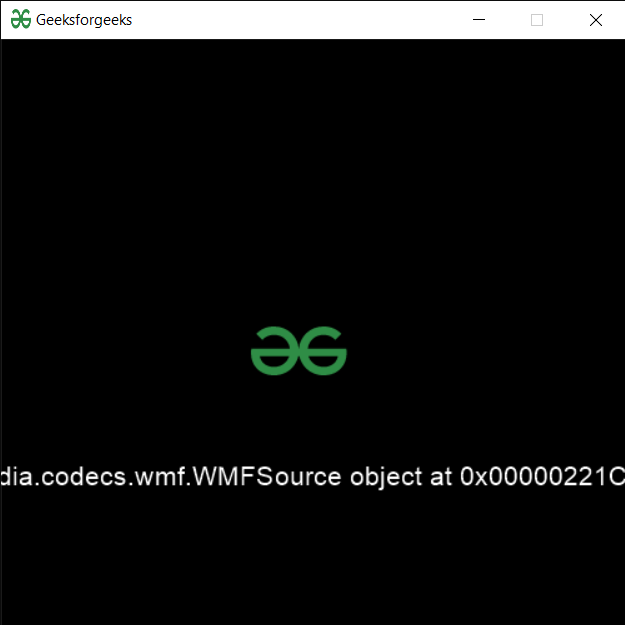• Last Updated : 06 Aug, 2021

In this article, we will see how we can load a media file in PYGLET module in python. Pyglet is easy to use but powerful library for developing visually rich GUI applications like games, multimedia, etc. A window is a “heavyweight” object occupying operating system resources. Windows may appear as floating regions or can be set to fill an entire screen (fullscreen). In order to load a file i.e resource we use resource module of pyglet. This module allows applications to specify a search path for resources. Relative paths are taken to be relative to the application’s __main__ module. Media is basically sound of video resource files

We can create a window object with the help of command given below

```# creating a window
window = pyglet.window.Window(width, height, title)```

In order to do this we use media method with the pyglet.resource
Syntax : resource.media(file_name)
Argument : It takes string i.e file name as argument
Return : It returns media.Source object

Below is the implementation

## Python3

 `# importing pyglet module``import` `pyglet``import` `pyglet.window.key as key``  ` `# width of window``width ``=` `500``  ` `# height of window``height ``=` `500``  ` `# caption i.e title of the window``title ``=` `"Geeksforgeeks"``  ` `# creating a window``window ``=` `pyglet.window.Window(width, height, title)``  ` `# text ``text ``=` `"Welcome to GeeksforGeeks"`` ` `# creating label with following properties``# font = cooper``# position = 250, 150``# anchor position = center``label ``=` `pyglet.text.Label(text,``                          ``font_name ``=``'Cooper'``,``                          ``font_size ``=` `16``,``                          ``x ``=` `250``, ``                          ``y ``=` `150``,``                          ``anchor_x ``=``'center'``, ``                          ``anchor_y ``=``'center'``)`  `# creating a batch``batch ``=` `pyglet.graphics.Batch()` `# loading geeksforgeeks image``image ``=` `pyglet.image.load(``'gfg.png'``)`  `# creating sprite object``# it is instance of an image displayed on-screen``sprite ``=` `pyglet.sprite.Sprite(image, x ``=` `200``, y ``=` `230``)``  ` `# on draw event``@window``.event``def` `on_draw():``      ` `    ``# clear the window``    ``window.clear()``      ` `    ``# draw the label``    ``label.draw()``    ` `    ``# draw the image on screen``    ``sprite.draw()``      ` `# key press event    ``@window``.event``def` `on_key_press(symbol, modifier):``  ` `    ``# key "C" get press``    ``if` `symbol ``=``=` `key.C:``        ` `        ``# printing the message``        ``print``(``"Key : C is pressed"``)``        ` `# image for icon``img ``=` `image ``=` `pyglet.resource.image(``"gfg.png"``)` `# setting image as icon``window.set_icon(img)` `# loading media file``value ``=` `pyglet.resource.media(``"media.mp4"``)` `# setting text  of label``label.text ``=` `str``(value)` `# start running the application``pyglet.app.run()`

Output :My Personal Notes arrow_drop_up